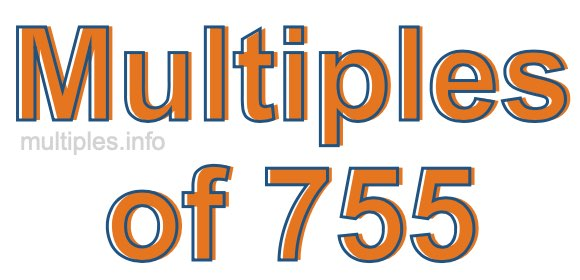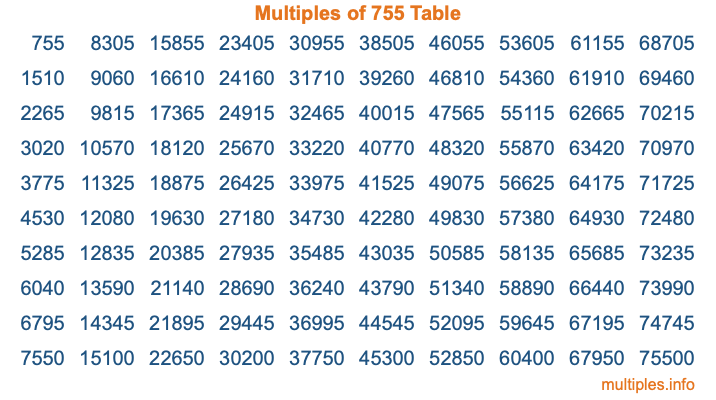Multiples of 755Welcome to the Multiples of 755 page. Here we will first teach you everything you will ever need to know about the multiples of 755, and then give you a study guide summary of everything we taught you to make sure you remember it all. Use this page to look up facts and learn information about the multiples of 755. This page will make you a multiples of seven hundred fifty-five expert!

Definition of Multiples of 755
Multiples of 755 are all the numbers that when divided by 755 equal an integer. Each of the multiples of 755 are called a multiple. A multiple of 755 is created by multiplying 755 by an integer.

Therefore, to create a list of multiples of 755, you start with 1 multiplied by 755, then 2 multiplied by 755, then 3 multiplied by 755, and so on for as long as you want. Thus, the list of the first five multiples of 755 is 755, 1510, 2265, 3020, and 3775. To see a larger list of multiples of 755, see the printable image of Multiples of 755 further down on this page. We also have a category where you can choose any nth multiple of 755.

Multiples of 755 Checker
The Multiples of 755 Checker below checks to see if any number of your choice is a multiple of 755. In other words, it checks to see if there is any number (integer) that when multiplied by 755 will equal your number. To do that, we divide your number by 755. If the the quotient is an integer, then your number is a multiple of 755.

Is  a multiple of 755?

Least Common Multiple of 755 and ...
A Least Common Multiple (LCM) is the lowest multiple that two or more numbers have in common. This is also called the smallest common multiple or lowest common multiple and is useful to know when you are adding our subtracting fractions. Enter one or more numbers below (755 is already entered) to find the LCM.

Check out our LCM Calculator if you need more details about the Least Common Multiple or if you need the LCM for different numbers for adding and subtraction fractions.

nth Multiple of 755
As we stated above, 755 is the first multiple of 755, 1510 is the second multiple of 755, 2265 is the third multiple of 755, and so on. Enter a number below to find the nth multiple of 755.

th multiple of 755

Multiples of 755 vs Factors of 755
755 is a multiple of 755 and a factor of 755, but that is where the similarities end. All postive multiples of 755 are 755 or greater than 755. All positive factors of 755 are 755 or less than 755.

Below is the beginning list of multiples of 755 and the factors of 755 so you can compare:

Multiples of 755: 755, 1510, 2265, 3020, 3775, etc.

Factors of 755: 1, 5, 151, 755

As you can see, the multiples of 755 are all the numbers that you can divide by 755 to get a whole number. The factors of 755, on the other hand, are all the whole numbers that you can multiply by another whole number to get 755.

It's also interesting to note that if a number (x) is a factor of 755, then 755 will also be a multiple of that number (x).

Multiples of 755 vs Divisors of 755
The divisors of 755 are all the integers that 755 can be divided by evenly. Below is a list of the divisors of 755.

Divisors of 755: 1, 5, 151, 755

The interesting thing to note here is that if you take any multiple of 755 and divide it by a divisor of 755, you will see that the quotient is an integer.

Multiples of 755 Table
Below is an image of the first 100 multiples of 755 in a table. The table is in chronological order, column by column. The first column has the first ten multiples of 755, the second column has the next ten multiples of 755, and so on.The Multiples of 755 Table is also referred to as the 755 Times Table or Times Table of 755. You are welcome to print out our table for your studies.

Negative Multiples of 755
Although not often discussed or needed in math, it is worth mentioning that you can make a list of negative multiples of 755 by multiplying 755 by -1, then by -2, then by -3, and so on, to get the following list of negative multiples of 755:

-755, -1510, -2265, -3020, -3775, etc.

Multiples of 755 Summary
Below is a summary of important Multiples of 755 facts that we have discussed on this page. To retain the knowledge on this page, we recommend that you read through the summary and explain to yourself or a study partner why they hold true.

There are an infinite number of multiples of 755.

A multiple of 755 divided by 755 will equal a whole number.

755 divided by a factor of 755 equals a divisor of 755.

The nth multiple of 755 is n times 755.

The largest factor of 755 is equal to the first positive multiple of 755.

755 is a multiple of every factor of 755.

755 is a multiple of 755.

A multiple of 755 divided by a divisor of 755 equals an integer.

755 divided by a divisor of 755 equals a factor of 755.

Any integer times 755 will equal a multiple of 755.

Multiples of a Number
Here you can get the multiples of another number, all with the same attention to detail as we did for multiples of 755 on this page.

Multiples of
Multiples of 756
Did you find our page about multiples of seven hundred fifty-five educational? Do you want more knowledge? Check out the multiples of the next number on our list!